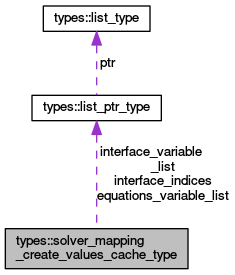OpenCMISS-Iron Internal API Documentation
types::solver_mapping_create_values_cache_type Type Reference

Contains information about the cached create values for a solver mapping. More...

Collaboration diagram for types::solver_mapping_create_values_cache_type:[legend]

## Public Attributes

type(list_ptr_type), dimension(:), pointer equations_variable_list
EQUATIONS_VARIABLES_LIST(solver_matrix_idx). The list of equations set variables in the solver mapping. More...

integer, dimension(:), allocatable dynamic_variable_type
DYNAMIC_VARIABLE_TYPE(equations_set_idx). The variable type that is mapped to the dynamic matrices for the equations_set_idx'th equations set. More...

integer(intg), dimension(:,:,:), allocatable matrix_variable_types
MATRIX_VARIABLE_TYPES(0:..,equations_set_idx,matrix_idx). The list of matrix variable types in the equations_set_idx'th equations set for the matrix_idx'th solver matrix. MATRIX_VARIABLE_TYPES(0,equations_set_idx,matrix_idx) is the number of variable types in the equations_set_idx'th equations set mapped to the matrix_idx'th solver matrix and MATRIX_VARIABLE_TYPES(1..,equations_set_idx,matrix_idx) is the list of the variable types in the equations set. More...

integer(intg), dimension(:,:), allocatable residual_variable_types
RESIDUAL_VARIABLE_TYPES(0:..,equations_set_idx). The list of residual variable types in the equations_set_idx'th equations set. RESIDUAL_VARIABLE_TYPES(0,equations_set_idx) is the number of variable types in the equations_set_idx'th equations set and RESIDUAL_VARIABLE_TYPES(1..,equations_set_idx) is the list of the variable types in the equations set. More...

integer(intg), dimension(:), allocatable rhs_variable_type
RHS_VARIABLE_TYPE(equations_set_idx). The variable type that is mapped to the solution RHS for the equations_set_idx'th equations set. More...

integer, dimension(:), allocatable source_variable_type
SOURCE_VARIABLE_TYPE(equations_set_idx). The source variable type that is mapped to the RHS for the equations_set_idx'th equations set. More...

type(list_ptr_type), dimension(:), pointer interface_variable_list
INTERFACE_VARIABLES_LIST(solver_matrix_idx). The list of interface condition variables in the solver mapping for the solver_matrix idx'th solver matrix. More...

type(list_ptr_type), dimension(:), pointer interface_indices
INTERFACE_INDICES(equations_set_idx). The list of interface condition indices in the equations_set_idx'th equations set. More...

## Detailed Description

Contains information about the cached create values for a solver mapping.

Definition at line 3058 of file types.f90.

## Member Data Documentation

 integer, dimension(:), allocatable types::solver_mapping_create_values_cache_type::dynamic_variable_type

DYNAMIC_VARIABLE_TYPE(equations_set_idx). The variable type that is mapped to the dynamic matrices for the equations_set_idx'th equations set.

Definition at line 3060 of file types.f90.

 type(list_ptr_type), dimension(:), pointer types::solver_mapping_create_values_cache_type::equations_variable_list

EQUATIONS_VARIABLES_LIST(solver_matrix_idx). The list of equations set variables in the solver mapping.

Definition at line 3059 of file types.f90.

 type(list_ptr_type), dimension(:), pointer types::solver_mapping_create_values_cache_type::interface_indices

INTERFACE_INDICES(equations_set_idx). The list of interface condition indices in the equations_set_idx'th equations set.

Definition at line 3066 of file types.f90.

 type(list_ptr_type), dimension(:), pointer types::solver_mapping_create_values_cache_type::interface_variable_list

INTERFACE_VARIABLES_LIST(solver_matrix_idx). The list of interface condition variables in the solver mapping for the solver_matrix idx'th solver matrix.

Definition at line 3065 of file types.f90.

 integer(intg), dimension(:,:,:), allocatable types::solver_mapping_create_values_cache_type::matrix_variable_types

MATRIX_VARIABLE_TYPES(0:..,equations_set_idx,matrix_idx). The list of matrix variable types in the equations_set_idx'th equations set for the matrix_idx'th solver matrix. MATRIX_VARIABLE_TYPES(0,equations_set_idx,matrix_idx) is the number of variable types in the equations_set_idx'th equations set mapped to the matrix_idx'th solver matrix and MATRIX_VARIABLE_TYPES(1..,equations_set_idx,matrix_idx) is the list of the variable types in the equations set.

Definition at line 3061 of file types.f90.

 integer(intg), dimension(:,:), allocatable types::solver_mapping_create_values_cache_type::residual_variable_types

RESIDUAL_VARIABLE_TYPES(0:..,equations_set_idx). The list of residual variable types in the equations_set_idx'th equations set. RESIDUAL_VARIABLE_TYPES(0,equations_set_idx) is the number of variable types in the equations_set_idx'th equations set and RESIDUAL_VARIABLE_TYPES(1..,equations_set_idx) is the list of the variable types in the equations set.

Definition at line 3062 of file types.f90.

 integer(intg), dimension(:), allocatable types::solver_mapping_create_values_cache_type::rhs_variable_type

RHS_VARIABLE_TYPE(equations_set_idx). The variable type that is mapped to the solution RHS for the equations_set_idx'th equations set.

Definition at line 3063 of file types.f90.

 integer, dimension(:), allocatable types::solver_mapping_create_values_cache_type::source_variable_type

SOURCE_VARIABLE_TYPE(equations_set_idx). The source variable type that is mapped to the RHS for the equations_set_idx'th equations set.

Definition at line 3064 of file types.f90.